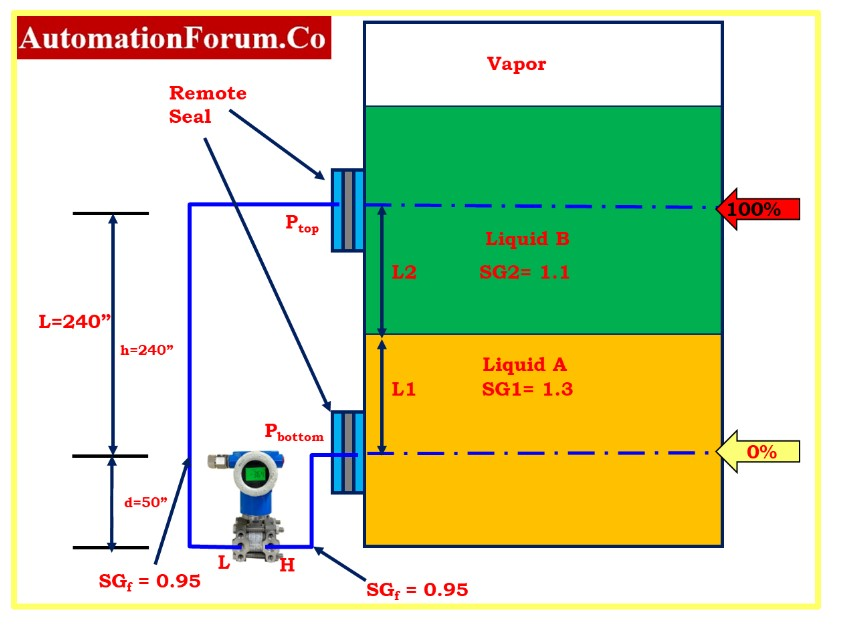# Interface level measurement using DP transmitter (Remote sealed)## How does DP transmitter measure interface level?

• In order to measure the interface of two fluids with various specific gravities(S), differential pressure (DP) transmitters are utilized.
• The overall level must always be at or above the low pressure tap in order to perform an interface measurement.
• The level must be large enough to produce a reasonable DP between the two extremes of specific gravity.
• Without or with remote seals, this measurement can be performed.
• However, from a maintenance perspective, using a remote seal assembly could be simpler; in certain situations, it might be challenging to maintain a consistent height for the wet leg.

## How do you measure interface level?

Example:

A vessel needs an interface measurement with a 240 inch level.

Two liquids with specific gravities of 1.1(SG2) and 1.3(SG1) are present in the container.

In order to calculate the calibrated range of the transmitter, four assumptions need to be made, and they are as follows:

1. The tank is filled with lighter fluid until it reaches the lower calibrated value, the 4 mA point.

2. The tank is filled with the heavier fluid until it reaches the upper calibrated value, 20 mA point.

3. The taps that go to the transmitter are constantly flooded. The total level must be at or above the upper (low pressure) tap.

4. The low pressure side may always observe a reference level. A wet leg or a remote seal system can be used to achieve this. The height and density of the reference level must remain constant. When the density of the high and low pressure wet legs is different, the calculation for wet leg systems is somewhat altered.

## How do you calculate URV and LRV?

The level that was measured is a combination of the two different fluids, which are as follows:

L = L1SG1 + L2SG2

H = The distance between taps.

L = Total Measured Level

S1 = Specific gravity of a heavier fluid

S2 = Lighter fluid’s specific gravity

Sf = Specific gravity of the reference leg (The type of transmitter is a capillary tube with a remote seal.)

Measured DP = The current DP value after transmitter calibration.

Span = The absolute difference between the maximum and minimum ranges.

LRV = Lower range value of the transmitter on the DP scale.

I = Interface level

## Calculation of interface measurement

When the tank is filled up to its full capacity with lighter fluid,

The transmitter will be set to 4 mA (or 0% of its span), and L2 = L:

HP = L2S2 + dSf

LP = dSf + hSf

At 4 mA,

DP = HP – LP

= (L2S2 + dSf)-(dSf + hSf)

= L2S2 – hSf

DP = (240 X 1.1) – (240 X 0.95)

=264 – 228

4 mA = 36 inH2O

The transmitter will be at 20 mA (or 100% of span) when the tank is full of the heavier fluid, and L1 will equal L because of this.

HP = L1S1 + dSf

LP = dSf + hSf

At 20 mA,

DP = HP – LP

= (L1S1 + dSf)-(dSf + hSf)

= L1S1 – hSf

DP = (240 x 1.3) – (240 x 0.95)

= 312 -228

20 mA = 84 inH2O

The calibration range is between 36 and 84 inH20.

When the transmitter reaches a reading of 36 inH20, the tank is considered to be completely full with lighter fluid.

When the transmitter reaches a reading of 84 inH20, the tank is considered to be full of the heavier fluid.

To establish the location of a fluid mixture’s interface, utilize the DP measurement as a percentage of span.

## How do you calculate interface level?

When the transmitter output is 18.4 mA or the DP is 20.7 inH2O, for instance, the interface level is determined using the following formula:

where, LCV= lower calibrated value

DP = Differential pressure indicated by the transmitter

Span = LRV – URV

L = Span Engineering range of the tank

Interface level I = {(77.55 – 36.00)/48} X 240

= (41.55 /48) X 240

Interface level I = 207.75 inches

Scroll to Top